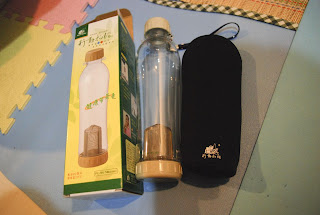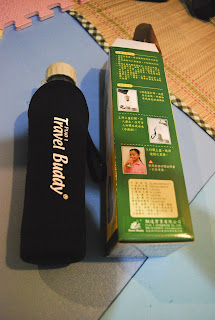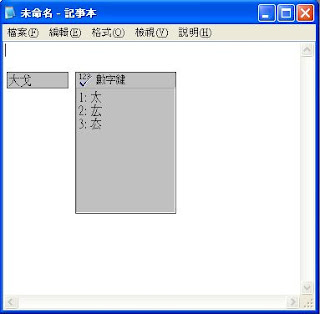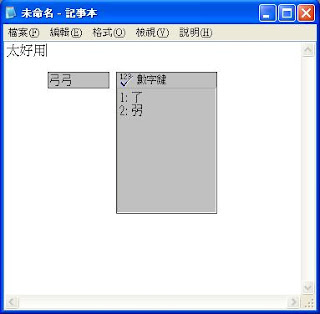## 2008年6月24日 星期二

### 將字串型態的運算式,轉成真正的數學運算式

Private Function CalculateEquation(ByVal strEquation As String) As Double
'要先引用 [Microsoft Script Control 1.0] 的 COM 元件
Dim MsScrCtl As New MSScriptControl.ScriptControl
MsScrCtl.Language = "VBSCript"
'傳回結果
Return sc.Eval(strNewEquation)
End Function

Public Class Form1

Private Structure typCalculateValue
Dim Name As String
Dim Value As Double
End Structure

Private Sub Button1_Click_1(ByVal sender As System.Object, ByVal e As System.EventArgs) Handles Button1.Click
Dim strValue(2) As typCalculateValue
strValue(0).Name = "TestA"
strValue(0).Value = 0.0894216
strValue(1).Name = "TestB"
strValue(1).Value = 0.0436392
strValue(1).Name = "TestC"
strValue(1).Value = 0.42
Dim strEquation As String
strEquation = "((TestA/2+(TestB*TestC)-123.456)/77)*(1e2)"
MsgBox(CalculateEquation(strValue, strEquation))
End Sub

Private Function CalculateEquation(ByVal aryTmpValue() As typCalculateValue, ByVal strEquation As String) As Double
'要先引用 [Microsoft Script Control 1.0] 的 COM 元件
Dim MsScrCtl As New MSScriptControl.ScriptControl
MsScrCtl.Language = "VBSCript"
Dim i As Integer
Dim strNewEquation As String
strNewEquation = strEquation
For i = 0 To UBound(aryTmpValue)
strNewEquation = Replace(strNewEquation, aryTmpValue(i).Name, aryTmpValue(i).Value)
Next
Return MsScrCtl.Eval(strNewEquation)
End Function
End Class

## 2008年6月22日 星期日

### 火狐(Firefox) 3好用套件_輕鬆升級

Tab Mix Plus

FlashGot
IE Tab
Super Drag and Go
Web Developer
.....等等的套件

http://quitt.net/mozilla/extensions/

## 2008年6月17日 星期二

### 桂花茶## 2008年6月8日 星期日

### 今年的第一口玉荷包## 2008年6月7日 星期六

### 真不巧,下雨了,無法照相## 2008年6月6日 星期五

### 輕盈的資料庫 SQLite

Command-Line程式也才幾百K,下載回來的DLL也一樣的小,

### TCL 和 SQLite 的應用

###=== 載入 下載回來的tclsqlite.DLL
###=== 建立新的資料庫
sqlite db1 F:/Andre_kao/testdb.db
db1 eval {CREATE TABLE t1(a int, b text)}
###=== 寫入資料
db1 eval {INSERT INTO t1 VALUES(1,'hello')}
db1 eval {INSERT INTO t1 VALUES(2,'goodbye')}
db1 eval {INSERT INTO t1 VALUES(3,'howdy!')}
set x [db1 eval
{SELECT * FROM t1 ORDER BY a}]
###--- 傳回 1 hello 2 goodbye 3 howdy!

###=== 將資料庫中的資料排序後印出來

db1 eval {SELECT * FROM t1 ORDER BY a} values {
parray values
puts ""
}
###=== 輸出結果
###=== values(*) = a b
###=== values(a) = 1
###=== values(b) = hello

###=== values(*) = a b
###=== values(a) = 2
###=== values(b) = goodbye

###=== values(*) = a b
###=== values(a) = 3
###=== values(b) = howdy!
###=== 請參考 http://www.sqlite.org/tclsqlite.html

### Metlab DLL 和 TCL 的應用

###### Metlab Code ######
###=== 建立一個 matlab 的檔案,命名為test2
###=== 程式碼如下

function [result] =test3(a,b,c,d)
result = a+b*c-d;
save('D:\MATLAB6p5\work');
return

###=== 在 Matlab 主程式中,選擇..
###=== Start --> Matlab --> Matlab COM Builder --> Matlab COM Builder
###=== 建立 New Project 取名為 test1
###=== Component Name 設為 testAAA
###=== 將 Build debug version 的選項打勾
###=== 執行Build產生 DLL 檔案
###=== 將DLL檔COPY到 C:\Windows\System32 裡面
################################

########### tcl 載入 DLL ###############

package require tcom
::tcom::import test1_1_0.dll
set comUtil [::test1::testAAA]
### 這裡的[::test1::testAAA]
### test1是Matlab 的COM Builder檔名
### testAAA是Matlab 的Component Name,也就是 Class Name

###===---  執行 DLL
### a 矩陣內容為
### { 1.0  2.0 }
### { 3.0  4.0 }

set a [list [list [expr double(1)] [expr double(2)]] [list [expr double(3)] [expr double(4)]] ]
### b 矩陣內容為
### { 3.0  2.0 }
### { 4.0  5.0 }

set b [list [list [expr double(3)] [expr double(2)]] [list [expr double(4)] [expr double(5)]] ]
### c 矩陣內容為
### { 1.0  2.0 }
### { 3.0  4.0 }

set c [list [list [expr double(1)] [expr double(2)]] [list [expr double(3)] [expr double(4)]] ]
### d 矩陣內容為
### { 4.0  5.0 }
### { 6.0  7.0 }

set d [list [list [expr double(4)] [expr double(5)]] [list [expr double(6)] [expr double(7)]] ]
set retx \$comUtil test2 1 retx \$a \$b \$c \$d
### 這裡的 \$comUtil test2 ....
### test2 是Matlab的M File Name,也就是存放程式碼的檔名

puts \$retx

### 上面的\$comUtil test2 1 retx.. "1" 表示傳回一個值 由 retx 變數接
### 傳回 {6.0 11.0} {16.0 25.0}

## 2008年6月5日 星期四

### 工程師節的禮物### Yahoo! 奇摩輸入法Yahoo! 奇摩輸入法### 教你如何用 Backy 收 Yahoo 的 pop3 信箱## 2008年6月4日 星期三

### 我口年的蚊帳### 使用 TCL 解高斯函數(矩陣 Matrix)

### 設定矩陣大小
### 矩陣內容如下
### MatrixA :
### |  1     1      2  |
### | 10    11   12 |
### MatrixB :
### |  5  |
### |  7  |

set X 3
set Y 2
### 引用 TCL 的數學函式 Library Package
package require
math::linearalgebra

### 建立矩陣
set MatrixA [::math::linearalgebra::mkMatrix \$Y \$X 0]
set MatrixB [::math::linearalgebra::mkMatrix \$Y 1 1]
### 填入矩陣 MatrixA 內容
::math::linearalgebra::setelem MatrixA 0 0 1
::math::linearalgebra::setelem MatrixA 0 1 1
::math::linearalgebra::setelem MatrixA 0 2 2
::math::linearalgebra::setelem MatrixA 1 0 10
::math::linearalgebra::setelem MatrixA 1 1 11
::math::linearalgebra::setelem MatrixA 1 2 12
### 填入矩陣 MatrixB 內容
::math::linearalgebra::setelem MatrixB 0 0 5
::math::linearalgebra::setelem MatrixB 1 0 7
### 使用 TCL 的 Library 計算 解高斯函數
set Z [::math::linearalgebra::solveGauss\$MatrixA \$MatrixB]
### 傳回答案 48.0 -43.0

### TCL中,將陣列(Array) 轉成不含Index 的 List 字串

### 取得陣列大小,如 Array1 的大小為 (3,5)
### 傳入 GetArraySize Array1 取得的值為 { 3 5 } 的 List
### 可由 [lindex [GetArraySize Array1] 0] 的方式取出 Row
###  [lindex [GetArraySize Array1] 1] 的方式取出 Col

proc
GetArraySize {arryName} {
set listName ""
upvar \$arryName arryNameII
set arrRowMax 0
set arrColMax 0
foreach {value} [array get arryNameII] {lappend arrElment \$value}
for {set i 1} {\$i = [llength \$arrElment]} {set i [expr \$i+2]} {
set arrIdx [lindex \$arrElment [expr \$i-1]]
set arrRow [string range \$arrIdx 0 [expr [string first "," \$arrIdx 0] - 1] ]
set arrCol [string range \$arrIdx [expr [string first "," \$arrIdx 0] + 1] [string length \$arrIdx]
]
if {\$arrRow>\$arrRowMax} {set arrRowMax \$arrRow}
if {\$arrCol>\$arrColMax} {set arrColMax \$arrCol}
}
return "\$arrRowMax \$arrColMax"
}

### 將陣列轉成List
### 因為用TCL的 array get 會連同 Array Index 同時轉成 List
### 所以另外寫一個 procedure ,將陣列內容轉成不含 Index 的 List
### 這一段才是主要的程轉換 Function

proc Array2List {srcArrName} {
upvar \$srcArrName srcArrNameII
### 呼叫副程式取出陣列的 Row 及 Col 大小
set srcArrNameII_r [lindex [GetArraySize srcArrNameII] 0]
set srcArrNameII_c [lindex [GetArraySize srcArrNameII] 1]
set destListName ""
for {set i 1} {\$i <= \$srcArrNameII_r} {incr i } {
set temp ""
for {set j 1} {\$j <= \$srcArrNameII_c} {incr j } {
lappend temp 0
}
lappend destListName \$temp
}
for {set i 1} {\$i <= \$srcArrNameII_r} {incr i } {
for {set j 1} {\$j <= \$srcArrNameII_c} {incr j } {
lset destListName [expr \$i-1] [expr \$j-1] [expr double (\$srcArrNameII(\$i,\$j))]
}
}
return \$destListName
}

## 2008年6月2日 星期一

### VB DLL 和 TCL 的應用

'################# VB DLL Class 設定 ###########
'### 開啟 VB 的 DLL 專案,並且命名為 ReCallDLL
'### 新增一個 Class 檔案 ,命名為 clsReCallDLL
'###---------- 加入以下程式碼 -----------------

Public Function testAB(a() As Variant, b()As Variant) As String
'組合第一個陣列內容
testAB = "aryStr = " & a(0) & b(0)
End Function

Public Function mathadd(a As Double, b As Double) As Double
'兩數相加
mathadd = a + b
End Function

Public Function mathadd2(a As Variant) As String
'傳回傳入字串
mathadd2 = "The Value is = " & a
End Function
'###----------------------------------------

################# TCL 的執行 Scrip ###########
### TCL 載入 DLL

package require tcom
::tcom::import ReCallDLL.dll     ;#採用動態(未安裝 DLL)載入
### 若是靜態(事先安裝DLL)載入則用下面的方法
#set comUtil [::ReCallDLL::clsReCallDLL]
### 建立連接端

set comUtil [::tcom::ref createobject "ReCallDLL.clsReCallDLL"]

### TCL 執行 DLL
set b "1 2"    ;#將 b 設為 List ,因為設成陣列Array 傳遞時會出現錯誤
set c "3 4"    ;#將 c 設為 List ,因為設成陣列Array 傳遞時會出現錯誤
set a [\$comUtil testAB b c]    ;#呼叫 DLL 並執行其中的一個 testAB Function
### 傳回 aryStr = 13
set b 12     ;#將 b 設為單一數字
set c 23    ;#將 c 設為單一數字
set a [\$comUtil mathadd bl cl] ;#呼叫 DLL 並執行 mathadd 將兩數相加並傳回
## 傳回 35

## 2008年6月1日 星期日

### nUtella 能多益榛子果仁可可醬

La buona prima colazione con nUtella